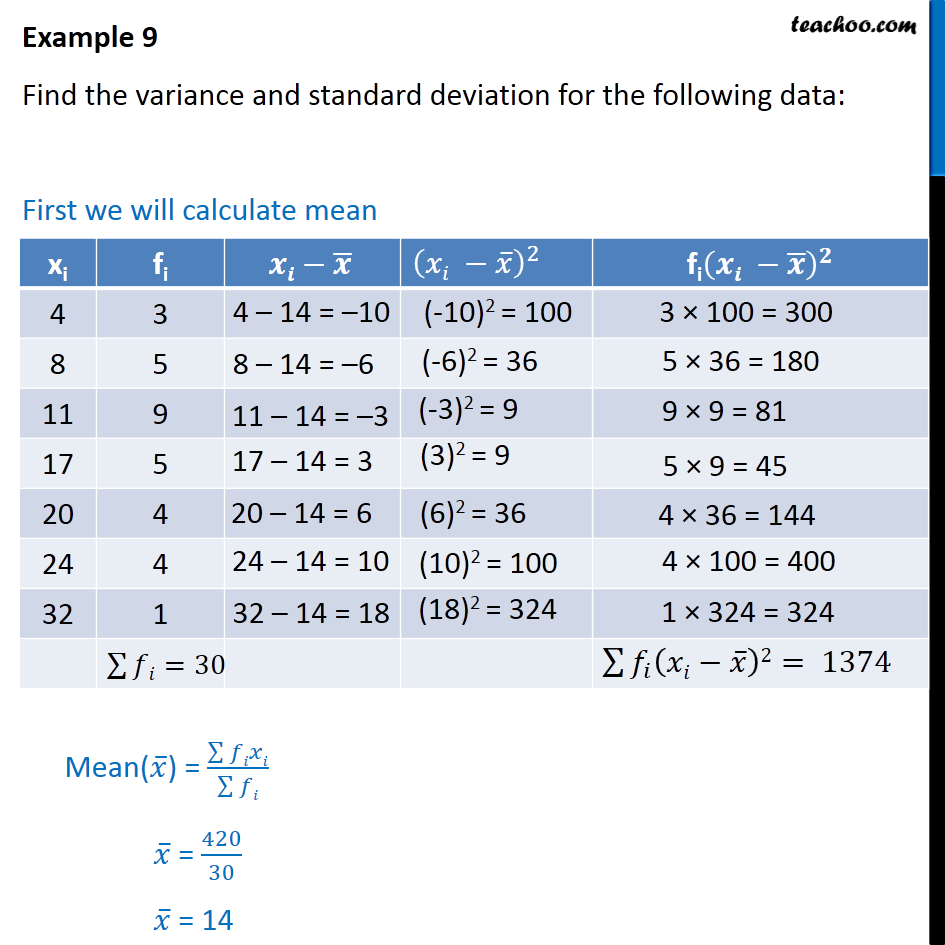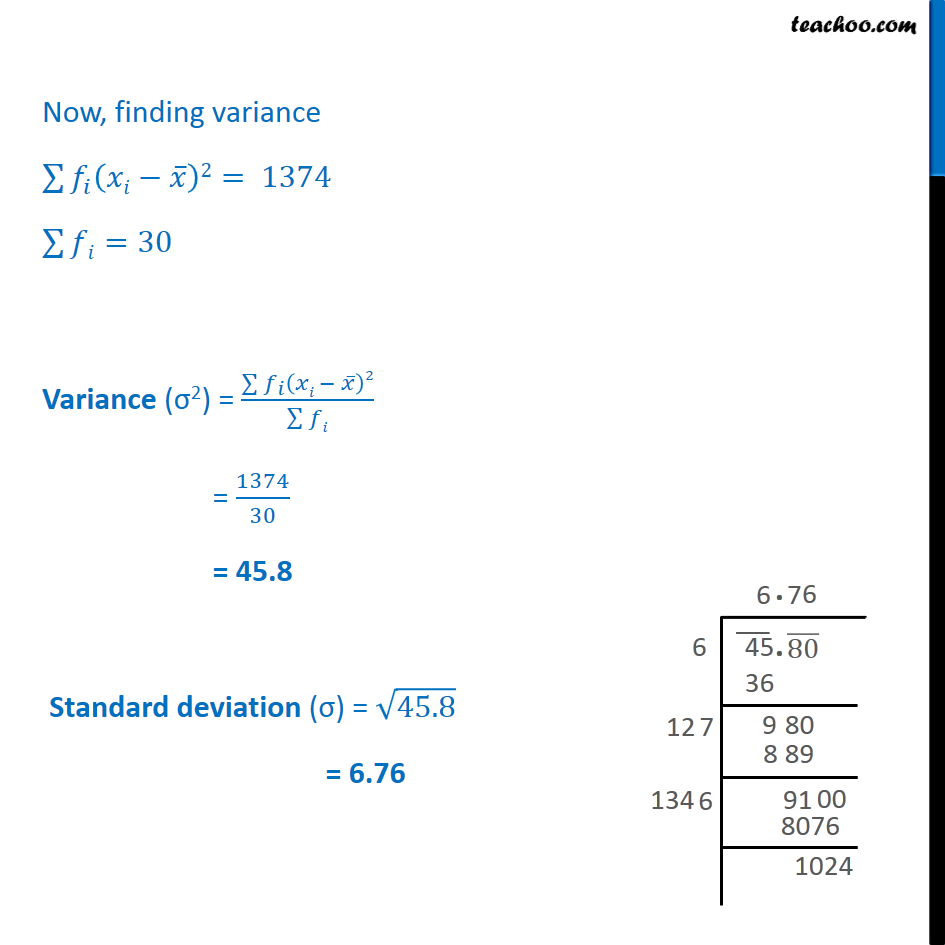Examples

Chapter 15 Class 11 Statistics
Serial order wiseMaths Crash Course - Live lectures + all videos + Real time Doubt solving!

### Transcript

Example 9 Find the variance and standard deviation for the following data: First we will calculate mean Now, finding variance 2 = 1374 =30 Variance ( 2) = 2 = 1374 30 = 45.8 Standard deviation ( ) = 45.8 = 6.76